EENS 2110 Mineralogy Tulane University Prof. Stephen A. Nelson Coordination and Pauling's Rules

 The arrangement of atoms in a crystal structure not only depends on the charge on the ion and type of bonding between atoms, but also on the size of the atoms or ions.  In any given molecule or crystal structure each atom or ion will be surrounded by other atoms or ions.  The number of  ions or atoms that immediately surround an atom or ion of interest is called the coordination number,  - C.N.  As we shall see, the coordination number depends on the relative size of the atoms or ions.  So, we must first discuss their sizes. Atomic and Ionic Radii The size of an atom or ion depends on the size of the nucleus and the number of electrons. Generally atoms with higher numbers of electrons have larger radii than those with smaller numbers of electrons.  Thus ions will have radii different from the atoms because ions will have either gained or lost electrons.  The number of positive charges in the nucleus determines both the number of electrons that surround an atom and the number of electrons that can be lost or gained to form ions.  Thus as the charge on the ion becomes more positive, there will be less electrons and the ion will have a smaller radius. As the charge on the ion becomes more negative, there will be more electrons and  the ion will have a larger radius. As the atomic number increases in any given column of the Periodic Table, the number of protons and electrons increases and thus the size of the atom or ion increases. Atomic and ionic radii also depend on the type of bonding that takes place between the constituents, and on the coordination number.  Thus, atomic and ionic radii will vary somewhat as a function of the environment in which the atoms or ions are found. A  list of ionic radii of the more common elements for various coordination numbers is shown on page 67 of your text.  First, let's examine the table looking at one column of the Periodic Table to see how the radii are affected by increasing atomic number.
Here we see the effect of increasing the atomic number (and total number of electrons) for ions of equal charge and the effect of changing the coordination number.  The radii increase with increasing total number of electrons downward in table.  Ionic radius also increases with increasing coordination number, the electron cloud is drawn out by the presence of more surrounding ions.
 Ion R(Å) C.N. = 6 R (Å) C.N. = 8 Li+1 0.74 0.92 Na+1 1.02 1.18 K+1 1.38 1.51 Rb+1 1.52 1.61 Cs+1 1.67 1.74

Next, we examine one row of the Periodic Table to see how the radii are affected by charge of the ion.

Here we see that as the charge becomes more positive the radius of the cation decreases.  This is because there are fewer electrons in the outer shells of the ions.  The sizes of anions are relatively large because there are more electrons in their outermost shells.

 Ion R(Å) C.N. = 4 R (Å) C.N. = 6 Na+1 0.99 1.02 Mg+2 0.57 0.72 Al+3 0.39 0.48 Si+4 0.26 0.40 P+5 0.17 0.38 S+6 0.12 0.29 S-2 1.84 Cl-1 1.81
 Coordination of Ions Coordination number, C.N.  depends on the relative size of the ions.  If all of the atoms in a crystal are the same size, then there are two ways to pack the atoms to form a crystal structure.  In this case, the maximum number of atoms that be coordinated around any individual is 12.  We call this 12-fold coordination.  There are two ways that atoms can be packed in 12-fold coordination.
 First, examine a single layer of atoms of equal size.  Note that there are two kinds of voids between the atoms, those that have a sort of triangular shape with the triangles pointing up we'll call B voids, and those with the triangles pointing down we'll call C voids. If we add the next layer of atoms so that they occupy the space above the B voids, and then add the next layer above the A atoms, this will result in a stacking sequence that runs AB AB AB ....etc.  This type of closest packing is referred to as hexagonal closest packing.  It results in a hexagonal lattice with the c-axis oriented perpendicular to the AB AB layers. If after adding the layer of B atoms we place the next layer so that the atoms occupy positions over the C voids in the A layer, and continue the process upward, we get a stacking sequence that runs ABC ABC ABC.... etc.  This type of packing is referred to as cubic closest packing.  It results in a cubic or isometric lattice with theaxis perpendicular to the layers.To see what happens when one of the involved ions or atoms becomes smaller, we need to examine the relative sizes of the atoms.  The relative sizes are indicated by the radius ratio of the coordinating atoms or ions.  In crystal structures we usually look at cations surrounded by anions, so the radius ratio is defined as Rx/Rz, where Rx is the radius of the cation, and Rz is the radius of the surrounding anions.  Since the anions are usually the larger ions, this results in decreasing values of Rx/Rz as the size of the cation decreases. If we decrease the size of the cation in such an arrangement, still allowing for the surrounding anions to touch each other and touch the cation, with decreasing size of the cation, the coordination will first result in 8 anions surrounding the cation.
 This is called 8-fold coordination or cubic coordination because the shape of the object constructed by drawing lines through the centers of the larger ions is a cube.   If the size of the coordinated cation becomes smaller, it will become too small  to touch the surrounding anions.  Thus, there is limiting radius ratio that will occur when the Rx/Rz becomes too small.  To see what this limit is, we must look at the vertical plane running through the centers anions labeled A and B.In this construction we can  determine the radius ratio for the limiting condition, often called the "no rattle limit" because if the radius ratio becomes smaller than this the cation will "rattle" in its site.  Using the Pythagorean theorem we can write:
 (2Rz +2Rx)2 = (2Rz)2 + (2Ö2Rz)2 2Rz +2Rx = √(4Rz2 + 8Rz2) 2Rz +2Rx = √(12Rz2) 2Rz +2Rx = 3.464Rz 2Rx = 1.464Rz Giving           Rx/Rz = 0.732Thus, for  Rx/Rz < 0.732 the cation will be too small or will rattle in its site and the structure will have to change to 6-fold coordination.
 Six-fold coordination is also called octahedral coordination because the shape defined by drawing planes through the center of the larger ions is an octahedron.  Octahedral coordination is stable when Rx/Rz , 0.732, but decreasing the radius of cation, Rx,  will eventually reach a limit where again the smaller ion will rattle in its site.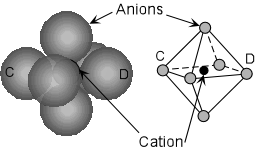The no rattle limit can be determined by looking at the horizontal plane running through the ions labeled C and D.  In this case we can write:
 (2Rz + 2Rx)2 = (2Rz)2 + (2Rz)2 = 2(4Rz2) 2Rz +2Rx = 2√2Rz Rz + Rx =  √2Rz Rx = (√2 - 1)Rz Rx/Rz = 0.414For Rx/Rz < 0.414 the structure goes into 4-fold coordination.  Planes through the centers of the larger atoms in this case will form a tetrahedron, so 4-fold coordination is also called tetrahedral coordination.The calculation to determine the "no rattle" limit for tetrahedral coordination is complex (see text by Klein and Dutrow, page  70).  The result shows that the limit is reached when Rx/Rz = 0.225.  As the radius ratio becomes smaller than this, triangular coordination becomes the stable configuration.
 For triangular coordination, the coordination number is three, that is 3 anions surround the smaller cation.  The "no rattle" limit is reached for triangular coordination when Rx/Rz becomes less than 0.155.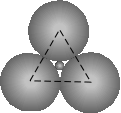At values of Rx/Rz < 0.155 the only way the smaller ion can be coordinated by the larger ions is to have 2 of the larger ions on either side.  This 2-fold coordination is termed linear coordination.The table here summarizes the cation to anion radius ratios, Rx/Rz, for various coordination numbers and gives the name of the coordination polyhedron for each coordination number.
 Rx/Rz C.N. Type 1.0 12 Hexagonal or Cubic Closest Packing 1.0 - 0.732 8 Cubic 0.732 - 0.414 6 Octahedral 0.414 - 0.225 4 Tetrahedral 0.225 - 0.155 3 Triangular <0.155 2 Linear
 In describing the structure of crystals and the locations of various ions or atoms within crystals, reference is often made to a crystallographic site on which an atom resides.  Such sites are usually referred to in terms of the coordination number or coordination polyhedron that surrounds the ion.  For example, in the silicate minerals Si is surrounded by 4 oxygens in tetrahedral coordination.  Thus Si is often said to occupy the tetrahedral sites in silicate minerals.  We will explore these concepts in more detail latter in the course.
 These general relationships of coordination only apply if the bonding is dominantly ionic.  In covalent structures the atoms overlap because they share electrons.  It should also be noted that 5-, 7-, 9-, and 10-fold coordination are also possible in complex structures. For the elements that occur in common minerals in the Earth's crust, the most common coordinating anion is Oxygen.  The following table gives the ionic radius and coordination of these common metal cations coordinated with oxygen.

 Ion C.N.  (with Oxygen) Coord. Polyhedron Ionic Radius,  Å K+ 8 - 12 cubic to closest 1.51 (8) - 1.64 (12) Na+ 8 - 6 cubic to octahedral 1.18 (8) - 1.02 (6) Ca+2 8 - 6 1.12 (8) - 1.00 (6) Mn+2 6 Octahedral 0.83 Fe+2 6 0.78 Mg+2 6 0.72 Fe+3 6 0.65 Ti+4 6 0.61 Al+3 6 0.54 Al+3 4 Tetrahedral 0.39 Si+4 4 0.26 P+5 4 0.17 S+6 4 0.12 C+4 3 Triangular 0.08

 Pauling's Rules Linus Pauling studied crystal structures and the types of bonding and coordination that occurs within them.  His studies found that crystal structures obey the following rules, now known as Pauling's Rules.Rule 1Around every cation, a coordination polyhedron of anions forms, in which the cation-anion distance is determined by the radius sums and the coordination number is determined by the radius ratio.This rule simply sets out what we have discussed above, stating that the different types of coordination polyhedra are determined by the radius ratio, Rx/Rz, of the cation to the anion.
 Rule 2, The Electrostatic Valency Principle  An ionic structure will be stable to the extent that the sum of the strengths of the electrostatic bonds that reach an ion equal the charge on that ion.In order to understand this rule we must first define electrostatic valency, e.v.e.v = Charge on the ion/C.N.
 For example, in NaCl each Na+ is surrounded by 6 Cl- ions.  The Na is thus in 6 fold coordination and C.N. = 6.  Thus e.v. = 1/6.  So 1/6 of a negative charge reaches the Na ion from each Cl.  So the +1 charge on the Na ion is balanced by 6*1/6 =1 negative charge from the 6 Cl ions.Similarly, in the CaF2 structure, each Ca+2 ion is surrounded by 8 F- ions in cubic or 8-fold coordination.  The e.v. reaching the Ca ion from each of the F ions is thus 1/4.  Since there are 8 F ions, the total charge reaching the Ca ion is 8*1/4 or 2.  So, again the charge is balanced.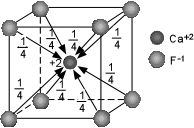Notice that in NaCl, each Cl ion is also surrounded by 6 Na ions in octahedral coordination.  So, again, the 1/6 of a positive charge from each Na reaches the Cl ion and thus the Cl ion sees 6*1/6 = 1 positive charge, which exactly balances the -1 charge on the Cl.   In the case of NaCl the charge is exactly balanced on both the cations and anions.  In such a case, we say that the bonds are of equal strength from all directions.  When this occurs the bonds are said to be isodesmic.This is not the case for C+4 ion in triangular coordination with O-2.  Here,  e.v. = 4/3 (C has a charge of +4 and is coordinated by 3 oxygens).  Thus, the 3 Oxygens each contribute 4/3 charge to the Carbon ion, and the charge on the carbon is balanced.  But, each Oxygen  still has 2/3 of a charge that it has not used.  Thus, a carbonate structural group is formed - CO3-2.   In cases like this, where the electrostatic valency is greater than 1/2 the charge on the anion (4/3 > 1/2*2), the anion will be more strongly bound to the central coordinating cation than it can be bonded to other structural groups.  When this occurs the bonding is said to be anisodesmic.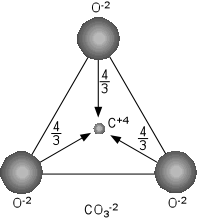A third case arises when the e.v. reaching the cation is exactly 1/2 the charge on the anion.  This is the case for Si+4 in tetrahedral coordination with O-2.  Here, the e.v. reaching the Si is 4/4 =1.  This leaves each Oxygen with a -1 charge that it has not shared.  Since this -1 is exactly 1/2 the original charge on O-2, the Oxygens in the SiO4-4 group can be just as tightly bound to ions outside the group as to the centrally coordinated Si.  In this case the bonding is said to be mesodesmic.   The SiO4-4 group is the basic building block of the most common minerals in the Earth's crust, the silicates.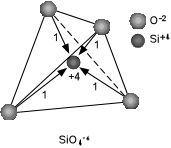Rule 3 Shared edges, and particularly faces of two anion polyhedra in a crystal structure decreases its stability.
 The reason for this is that sharing of only corners of  polyhedra places the positively charged cations at the greatest distance from each other.  In the example shown here, for tetrahedral coordination, if the distance between the cations in the polyhedrons that share corners is taken as 1, then sharing edges reduces the distance to 0.58, and sharing of faces reduces the distance to 0.38.Rule 4 In a crystal structure containing several cations, those of high valency and small coordination number tend not  to share polyhedral elements. Sharing of polyhedral elements for cations of high charge will place cations close enough together that they may repel one another.  Thus, if they do not share polyhedral elements they can be better shielded from the effects of other positive charges in the crystal structure. Rules 1 through 4 maximize the cation - anion attractions and minimize the anion-anion and cation-cation repulsions. Rule 5, The Principle of Parsimony The number of different kinds of constituents in a crystal tends to be small.   This means that there are only a few different types of cation and anion sites in a crystal. Even though a crystal may have tetrahedral sites, octahedral sites, and cubic sites, most crystals will be limited to this small number of sites, although different elements may occupy similar sites. Next time we will see how these principles apply to the silicate minerals.
 Examples of questions on this material that could be asked on an exam Define the following: (a) coordination number, (b) octahedral coordination, (c) tetrahedral coordination, (d) tringular coordination, (e) electrostatic valence, (f) isodesmic, (g) anisodesmic, (h) mesodesmic. Explain what factors control the size of ions and why. Why are anions in general larger than cations? Expalin Pauling's five rules in simple terms and explain why they are important. Return to EENS 2110 Page